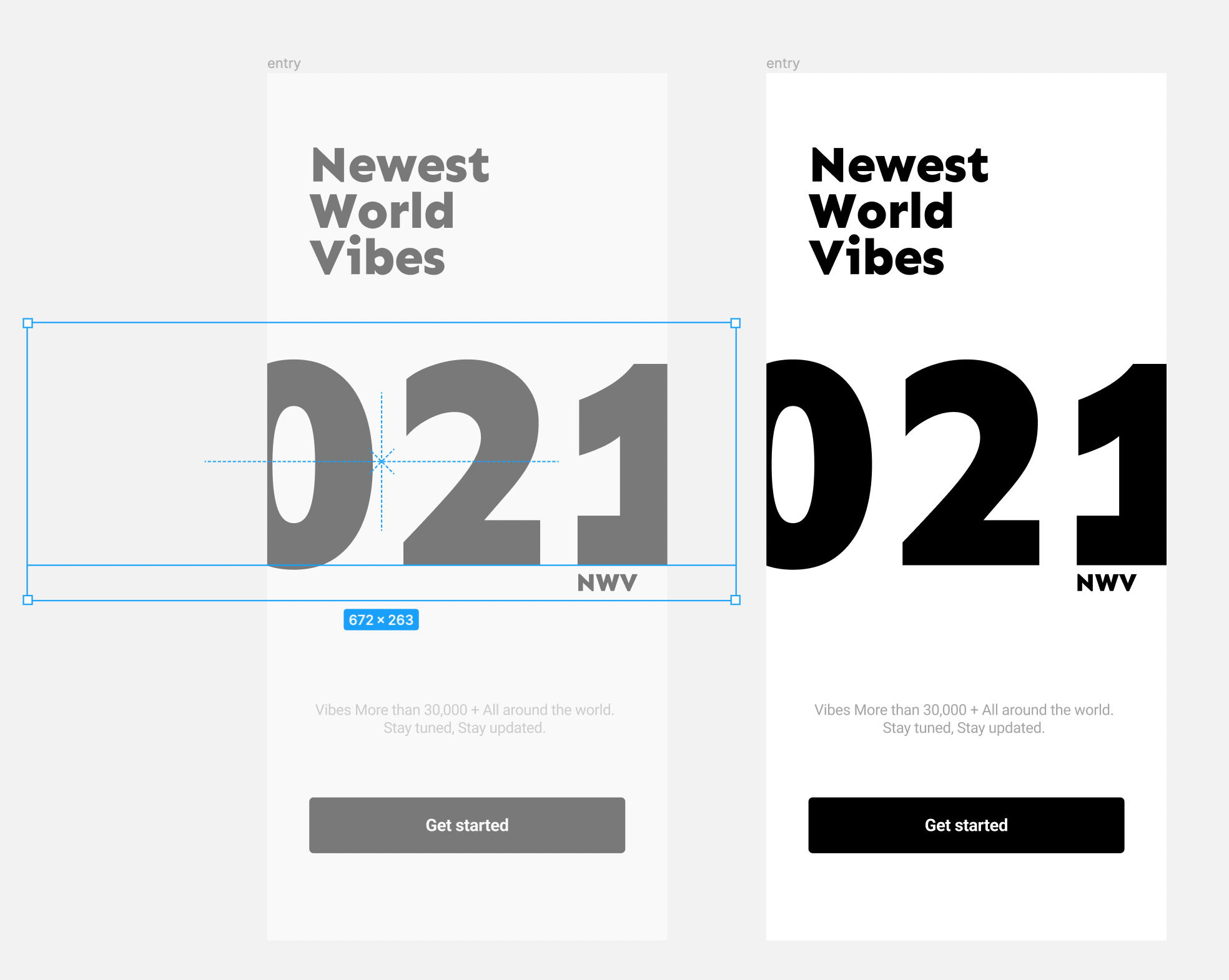# Item overflowing scenarios (static, no-scroll)

This is not a document for overflowing layout, a scroll. (Go here for overflowing layout scroll docs)

This document describes how to handle intentionally overflowed items per by frameworks.

This is a good example of the overflowing static example.## Web - css​

``.container {  background-color: black; /*(visual factor)*/  overflow: hidden; /* <-- do not scroll overflow */}.overflow {  position: relative;  left: calc(    (100% - 1000px) * 0.5  ); /* <-- overflow static position (center) (parent.width - this.with / 2) */  /* or for non-semitric alignment, left:calc((100% - w - x) / 2) */  margin: 10px; /*(visual factor)*/  background-color: red; /*(visual factor)*/  width: 1000px; /* <-- overflowing size */}``

The math for finding the constrainted position is.

• left: `calc((100% - w - x) / 2)`

## Flutter​

Using OverflowBox

``OverflowBox(    minWidth: 0.0,    minHeight: 0.0,    maxWidth: double.infinity,    maxHeight: double.infinity,    alignment: Alignment.center,    /* <--- we can dynamically align to match the design's snapshot */    child:        Container(            color: red,            width: 4000,            /* <--- overflowing width */            height: 50        ));``

The math for finding the constrainted position is.

• alignment: `Alignment(x, y)`

References